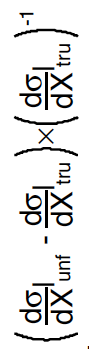# Latex on axis label for "evaluated at" symbol

Hello,

I would like to use the symbol | in an axis label, specifically in this way:

#frac{d#sigma}{dp_{T}^{top}}|_{tru}”

However, the vertical line doesn’t cover the whole height of the fraction. In latex you would typically do:

#frac{d#sigma}{dp_{T}^{top}}#right|_{tru}”

or

#frac{d#sigma}{dp_{T}^{top}}#bigg|_{tru}”

but in ROOT if I use “#right” without a “#left” it fails giving an error and if I use “#bigg” it just prints “#bigg” in the axis label.

Is there some other way?

Thank you.Since ROOT version v5-34-04 you can do:

“\frac{d\sigma}{dp_{T}^{top}}|_{tru}”